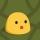Home
IT Knowledge
Inspiration
Languages
EN

# Python - functions

0 points
Created by:Molly
201

In this article, we would like to show you how to create and call a function in Python.

Quick solution:

``````def my_function():
print("Hello World!")

my_function()  # Hello World!``````

## 1. Create function

In the Quick solution we presented how to create a function without arguments.

In this example, we create a function that takes 2 arguments separated by a comma (`a``b`) and returns the sum of them.

``````def my_function(a, b):
return a + b``````

## 2. Call function

You can call a function by typing the function name with the parenthesis. If your function takes some arguments, you need to pass them when the function is called (in our example `a` = `1`, `b` = `2`).

``````def my_function(a, b):
return a + b

result = my_function(1, 2)
print("result =", result)``````

Output:

``result = 3``